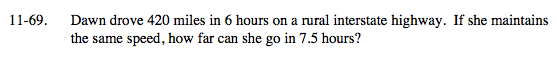### Home > MC2 > Chapter 11 > Lesson 11.2.4 > Problem11-69

11-69.Since Dawn will maintain the same speed, we can find the equivalent fraction for 7.5 hours to solve for the distance.

$\frac{\text{miles}}{\text{hour}} = \frac{420}{6} = \frac{\it x}{7.5}$

$70 = \frac{\it x}{70}$

x = 525 miles